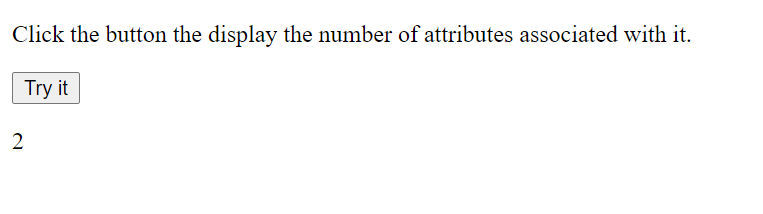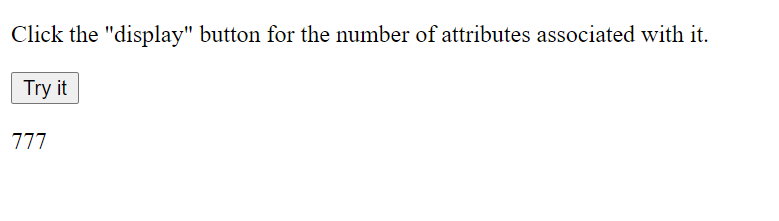Related Articles
What is the difference between properties and attributes in HTML?
• Last Updated : 17 Mar, 2021

HTML is the standard language for creating documents that are intended to be displayed on web browsers. It is very usual to get confused with attributes and properties while performing DOM object manipulation in JavaScript. Before learning about the differences, let us first define the Document Object Model.

DOM: It is the acronym for the Document Object Model. When the browser parses your HTML code, it creates a corresponding DOM node. The HTML properties are accessed from this node object.

In simpler terms, DOM is an API for the HTML, and we use languages like JavaScript or frameworks like React, Vue.js to access and manipulate the HTML using the corresponding DOM objects.

Notes:

1. Some DOM properties don’t possess corresponding attributes.
2. Some HTML attributes don’t possess corresponding properties.
3. Some HTML attributes, like ‘class’, possess 1:1 mapping to properties.

Let us take a look at some quick examples to demonstrate the differences between attributes and properties.

Example: Consider the following HTML code snippet.

## html

 ```<``html``>` `<``body``>``    ``<``input` `id``=``"input"` `type``=``"number"``            ``value``=``"Phone Number:"``>` `    ``<``p` `id``=``"display"``>`  `    ``<``script``>``        ``var element = document.getElementById("input");` `        ``// Getting the property, returns "Phone Number:"``        ``window.alert(element.getAttribute("value"));` `        ``element.value = 1234;``        ``var x = element.value;` `        ``// Getting the attribute, returns "1234"``        ``document.getElementById("display").innerHTML``            ``= "Value Attribute: " + x;``    ````` `         `

Output:Now, let us consider that the user inputs “1234”, then the element.getAttribute(“value”) will return “Phone Number:” because we have provided this as the initial value of this attribute. But element.value will return “1234”.

Thus, the value attribute has the initial text-content that was provided by the developer in the HTML source code because the value property reflects the current text-content inside the input box (provided by the user in this case).

Now we have a basic idea about the difference, let us dive deep and learn about more differences.

Attribute: Attributes are defined by HTML and are used to customize a tag.

## html

 ```<``html``>` `<``body``>` `    ` `<``p``>``        ``Click the button the display``        ``the number of attributes``        ``associated with it.``    ``` `    ` `    ``<``button` `id``=``"AttributeDemo"``        ``onclick``=``"myFunction()"``>``        ``Try it``    ````    ` `    ``<``p` `id``=``"display"``>`  `    ``<``script``>``        ``function myFunction() {``            ``var Attrdemo = document.getElementById(``                ``"AttributeDemo").attributes.length;` `            ``document.getElementById(``                ``"display").innerHTML = Attrdemo;``        ``}``    ````` ``The output is 2 because the two attributes are: id=”AttributeDemo” and onclick=”myFunction()”.

Note: The document.getElementsById(‘AttributeDemo’).attributes code snippet returns a collection of the specified node’s attributes, as a NamedNodeMap object. To access the nodes, we can use the generic indexing method. Replace the following line in the above code snippet.

## javascript

 `var` `Attrdemo = document.getElementById(``    ``'AtrributeDemo'``).attributes.name;`

Output:

`onclick`

The attributes have a data type of string. So no matter the value of the attribute, it will always return a string.
Example:

`document.getElementById('AtrributeDemo').getAttribute('id')`

Output:

`AttributeDemo`

Property: In contrast to the attributes, which are defined in HTML, properties belong to the DOM. Since DOM is an object in JavaScript, we can get and set properties.

## javascript

 ```` `` `    ` `

``        ``Click the ``"display"` `button ``for``        ``the number of attributes``        ``associated ``with` `it.``    ``

`  `    ``` `    ``

`  `    ````` ``

Output:Default attributes (non-user-defined) change when corresponding property changes and vice-versa.

## html

 ```<``html``>` `<``body``>` `    ` `<``p``>``        ``Click the button the display the``        ``current className & the edited``        ``className``    ``` `    ` `    ``<``button` `id``=``"demo1"` `class``=``"button"``        ``onclick``=``"Function1()"``>``        ``Click Me``    ````    ` `    ``<``p` `id``=``"displayCurrent"``>` `    ``<``p` `id``=``"diplayEdited"``>` `    ` `    ``<``script``>``        ``function Function1() {``            ``var div = document.getElementById("demo1");` `            ``// Returns  "GeeksForGeeks"``            ``window.alert("Current Class : "``                ``+ div.getAttribute("class"));` `            ``div.setAttribute("class", "GeeksForGeeks");` `            ``// Returns : "GeeksForGeeks"           ``            ``window.alert("Edited Class : "``                ``+ div.getAttribute("class"));``        ``}``    ````` ``

Output:Note:

1. Non-custom attributes (like id, class, etc.) have 1:1 mapping to the properties.
2. We use ‘className’ to access (get or set) the ‘class’ property because ‘class’ is a reserved keyword in JavaScript.
3. Attributes that have a defined default value remain constant when the corresponding property changes.

Difference between HTML attributes and DOM properties:

My Personal Notes arrow_drop_up